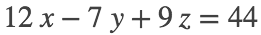# MATH 251: Calculus 3, SET8

## 14: Partial Derivatives

### 14.4: Tangent Planes and Linear Approximation

These problems are done with the CAS. See Hand Solutions for details.

#### 1. [934/2]

syms x y z
f(x,y) = (x+2)^2 - 2*(y-1)^2 - 5
f(x, y) =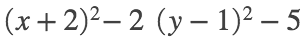fx = diff(f,x), fy = diff(f,y)
fx(x, y) =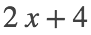fy(x, y) =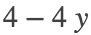our_surface = z == f(x,y)
our_surface =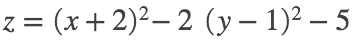P = [2 3 f(2,3)] % check
P =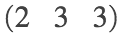fxP = fx(2,3), fyP = fy(2,3)
fxP = 8
fyP =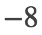eq = z - 3 == fxP*(x-2) + fyP*(y-3)
eq =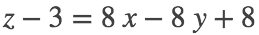tangent_plane_at_P = eq + 3
tangent_plane_at_P =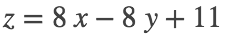#### 2. [934/6]

syms x y z
f(x,y) = log(x-2*y)
f(x, y) =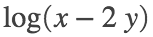fx = diff(f,x), fy = diff(f,y)
fx(x, y) =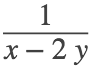fy(x, y) =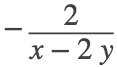our_surface = z == f(x,y)
our_surface =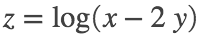P = [3 1 f(3,1)] % check
P =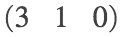fxP = fx(3,1), fyP = fy(3,1)
fxP = 1
fyP =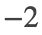tangent_plane_at_P = z - 0 == fxP*(x-3) + fyP*(y-1)
tangent_plane_at_P =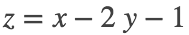#### 3. [934/8]

syms x y z
f(x,y) = sqrt(9 + x^2*y^2)
f(x, y) =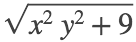fx = diff(f,x), fy = diff(f,y)
fx(x, y) =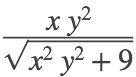fy(x, y) =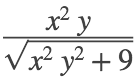our_surface = z == f(x,y)
our_surface =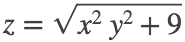P = [2 2 f(2,2)] % check
P =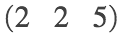fxP = fx(2,2), fyP = fy(2,2)
fxP =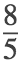fyP =eq = z - 5 == fxP*(x-3) + fyP*(y-1)
eq =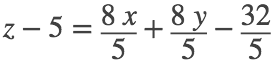tangent_plane_at_P = eq + 5
tangent_plane_at_P =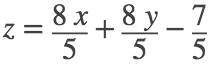%
figure
fsurf(f(x,y), [-3 3 -3 3], 'MeshDensity', 20); hold on
fsurf(rhs(tangent_plane_at_P), [0 3 0 3], 'm', 'MeshDensity', 10)
plot3(2,2,5, 'bo', 'MarkerFaceColor', 'k', 'MarkerSize', 20)
view(39,36)
xlabel('x'); ylabel('y'); zlabel('z')
title('SET8, 934/8')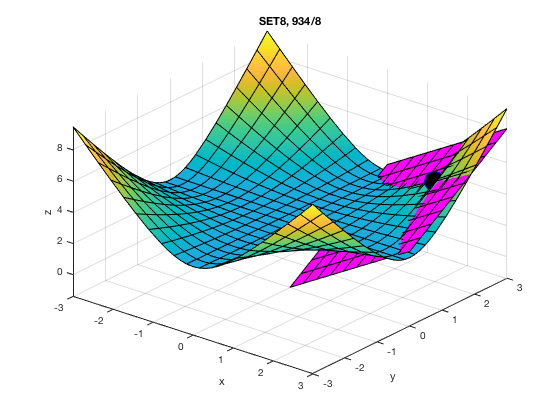#### 4. [934/14]

Note that the partial derivatives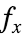and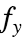exist at and are continuous near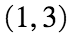. Therefore, f is differentiable by Theorem 8 on page 931. Hence the linearization L of f exists and is computed below.
syms x y
f(x,y) = (1+y) / (1+x)
f(x, y) =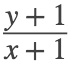fx = diff(f,x), fy = diff(f,y)
fx(x, y) =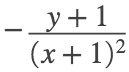fy(x, y) =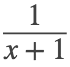fxP = fx(1,3), fyP = fy(1,3)
fxP =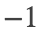fyP =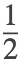L(x,y) = f(1,3) + fxP*(x-1) + fyP*(y-3)
L(x, y) =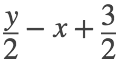#### 5. [935/20]

We see that the value of the linearization is close to that of the function nearby.
syms x y
f(x,y) = 1 - x*y*cos(pi*y)
f(x, y) =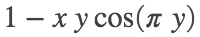fx = diff(f,x), fy = diff(f,y)
fx(x, y) =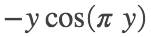fy(x, y) =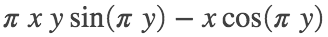fxP = fx(1,1), fyP = fy(1,1)
fxP = 1
fyP = 1
L(x,y) = f(1,1) + fxP*(x-1) + fyP*(y-1)
L(x, y) =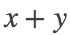fP = double(f(1.02, 0.97)) % function value
fP = 1.9850
LP = double(L(1.02, 0.97)) % linear appx val
LP = 1.9900

#### 6. [935/30]

We take a page off the Section 12.6 playbook and employ the gradient.
It computes the three first-order partial derivatives of f all at once.
MATLAB jumbles the order of the summands of the differential df. See Hand Solutions for a cleaner version.
syms dx dy dz x y z real
f = x*z*exp(-y^2 - z^2)
f =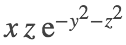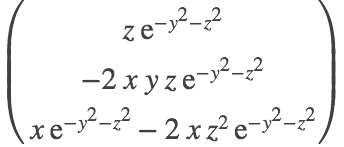df = dot(grad_f, [dx dy dz]) % the differential df
df =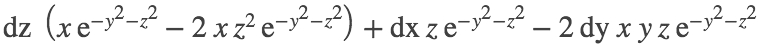#### 7. [935/32]

syms dx dy x y real
z(x,y) = x^2 - x*y + 3*y^2, P = sym([3 1])
z(x, y) =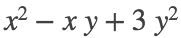P = 1×2
3 1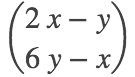dz(x,y,dx,dy) = dot(grad_z(x,y), [dx dy]) % the differential dz
dz(x, y, dx, dy) =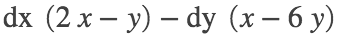delta_z = double(z(2.96, -0.95) - z(3, -1)) % Notice the change in z along surface
delta_z = -0.7189
dz_at_P = double(dz(3, -1, 2.96-3, -0.95-(-1))) % is close to that along tangent plane.
dz_at_P = -0.7300

#### 8. [935/34]

syms dh dr h r real
V(h,r) = pi*r^2*h, P = sym([10 2])
V(h, r) =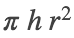P =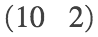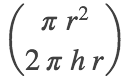dV(h,r,dh,dr) = dot(grad_V(h,r), [dh dr]) % the differential dV
dV(h, r, dh, dr) =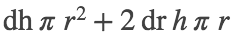appx_vol = double(dV(10, 2, 2*0.1, 0.05)) % in cm^3
appx_vol = 8.7965
% NOTE WELL: Here dh = 2*0.1, NOT 0.1, to account for TOP and BOTTOM lids!

#### 9. [935/38]

Compute the pressure differential. Evaluate it using the starting temperature and volume and changes in same.
syms dT dV T V real
P(T,V) = 8.31*T/V
P(T, V) =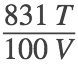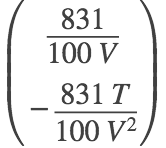dP(T,V,dT,dV) = dot(grad_P(T,V), [dT dV]) % the differential dP
dP(T, V, dT, dV) =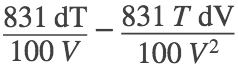appx_pressure_chg = double(dP(310, 12, -5, 0.3)) % in kPa (kilopascals)
appx_pressure_chg = -8.8294

#### 10. [936/42]

Compute tangent vectors to the curves at the point. Their cross product is a normal vector to the plane at the point. From this we can construct an equation of the tangent plane.
syms t u x y z
r1(t) = [2+3*t 1-t^2 3-4*t+t^2]
r1(t) =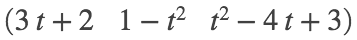r2(u) = [1+u^2 2*u^3-1 2*u+1]
r2(u) =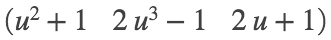P = r1(0), P = r2(1) % The point is on both curves.
P =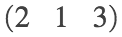P =Dr1(t) = diff(r1,t), Dr2(u) = diff(r2,u)
Dr1(t) =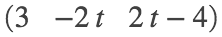Dr2(u) =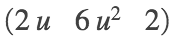v = Dr1(0), w = Dr2(1) % tangent vectors to curves at P
v =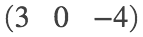w =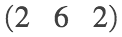n = cross(v,w) % normal vector to tangent plane to surface at P
n =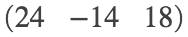eq = dot(n,[x y z]) == dot(n,P)
eq =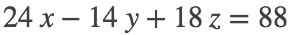tangent_plane = eq/2
tangent_plane =